# Viacheslav Borovitskiy (Slava)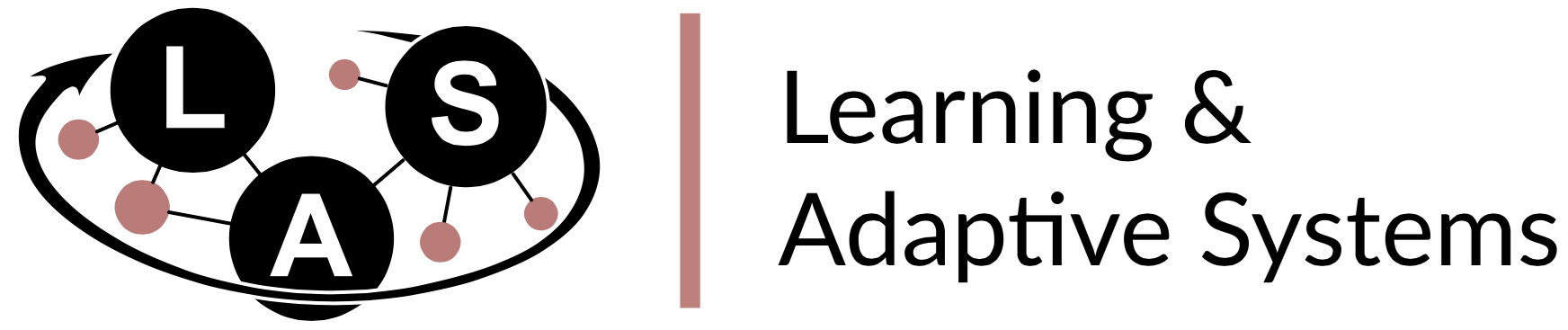# Gaussian Processes

Definition. A Gaussian process is random function $f : X \times \Omega \to \R$ such that for any $x_1,..,x_n$, the vector $f(x_1),..,f(x_n)$ is multivariate Gaussian.

The distribution of a Gaussian process is characterized by

• a mean function $m(x) = \E(f(x))$,
• a kernel (covariance) function $k(x, x') = \Cov(f(x), f(x'))$,

Notation: $f \~ \f{GP}(m, k)$.

The kernel $k$ must be positive (semi-)definite, i.e. for all $x_1, .., x_n \in X$
the matrix $K_{\v{x} \v{x}} := \cbr{k(x_i, x_j)}_{\substack{1 \leq i \leq n \\ 1 \leq j \leq n}}$ must be positive (semi-)definite.

# Gaussian Process Regression

Takes

• a prior Gaussian Process $\f{GP}(m, k)$
• and data $(x_1, y_1), .., (x_n, y_n) \in X \x \R$,

giving the posterior (conditional) Gaussian process $\f{GP}(\hat{m}, \hat{k})$.

The functions $\hat{m}$ and $\hat{k}$ may be explicitly expressed in terms of $m$ and $k$.

# An Application: Bayesian Optimization

Goal: minimize unknown function $\phi$ in as few evaluations as possible.

1. Build GP posterior $f \given \v{y}$ using data $(x_1,\phi(x_1)),..,(x_n,\phi(x_n))$.
2. Choose $$\htmlClass{fragment}{ x_{n+1} = \argmax_{x\in\c{X}} \underbrace{\alpha_{f\given\v{y}}(x).}_{\text{acquisition function}} }$$ For instance, expected improvement $$\alpha_{f\given\v{y}}(x) = \E_{f\given\v{y}} \max(0, {\displaystyle\min_{i=1,..,n}} \phi(x_i) - f(x)).$$

# Bayesian Optimization# Applications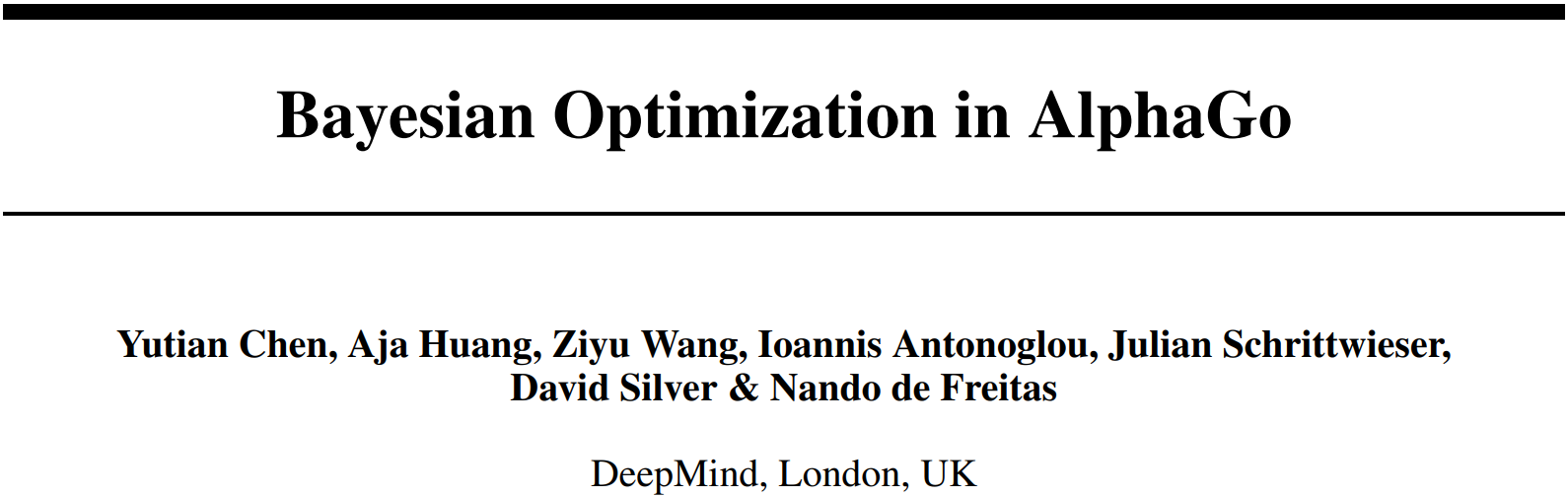Also

• geostatistics,
• robotics,
• more...

# Matérn Gaussian Processes

$$\htmlData{class=fragment fade-out,fragment-index=9}{ \footnotesize \mathclap{ k_{\nu, \kappa, \sigma^2}(x,x') = \sigma^2 \frac{2^{1-\nu}}{\Gamma(\nu)} \del{\sqrt{2\nu} \frac{\norm{x-x'}}{\kappa}}^\nu K_\nu \del{\sqrt{2\nu} \frac{\norm{x-x'}}{\kappa}} } } \htmlData{class=fragment d-print-none,fragment-index=9}{ \footnotesize \mathclap{ k_{\infty, \kappa, \sigma^2}(x,x') = \sigma^2 \exp\del{-\frac{\norm{x-x'}^2}{2\kappa^2}} } }$$ $\sigma^2$: variance $\kappa$: length scale $\nu$: smoothness
$\nu\to\infty$: Gaussian kernel (RBF)$\nu = 1/2$$\nu = 3/2$$\nu = 5/2$$\nu = \infty$

# Non-Euclidean Domains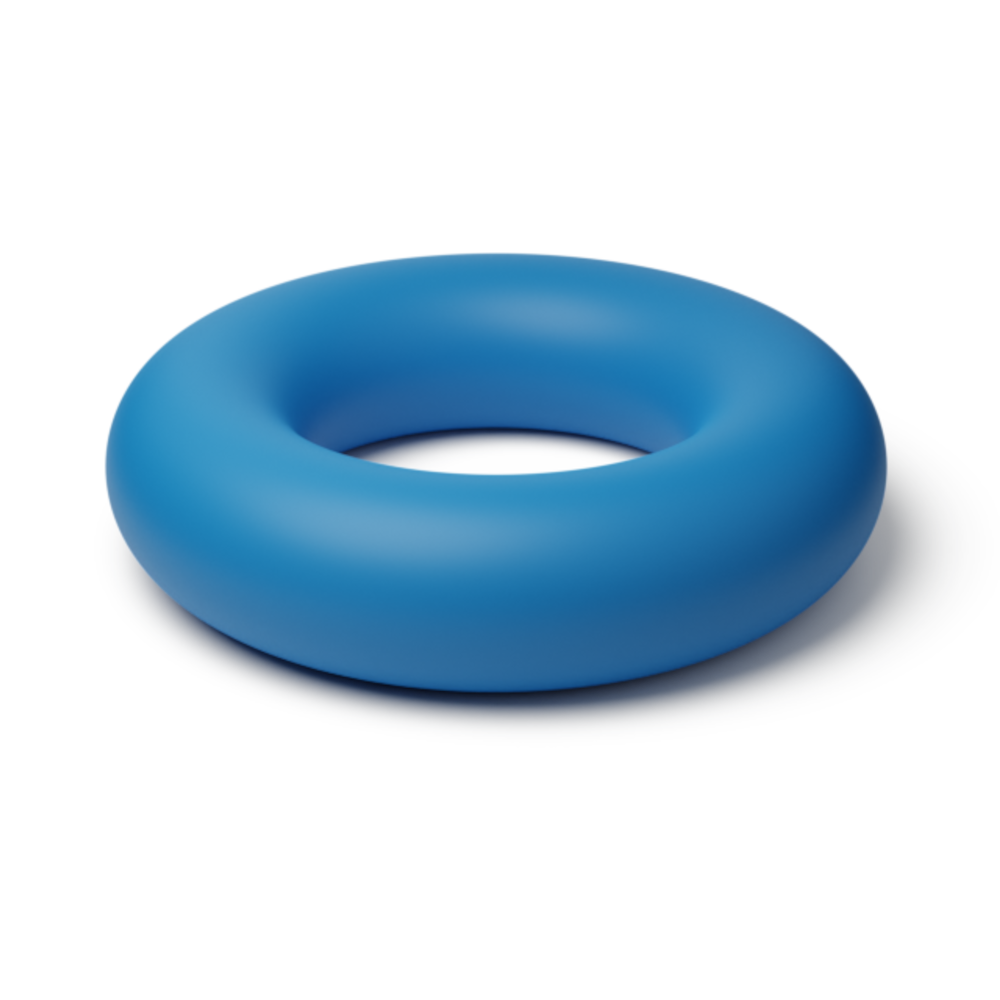### Manifolds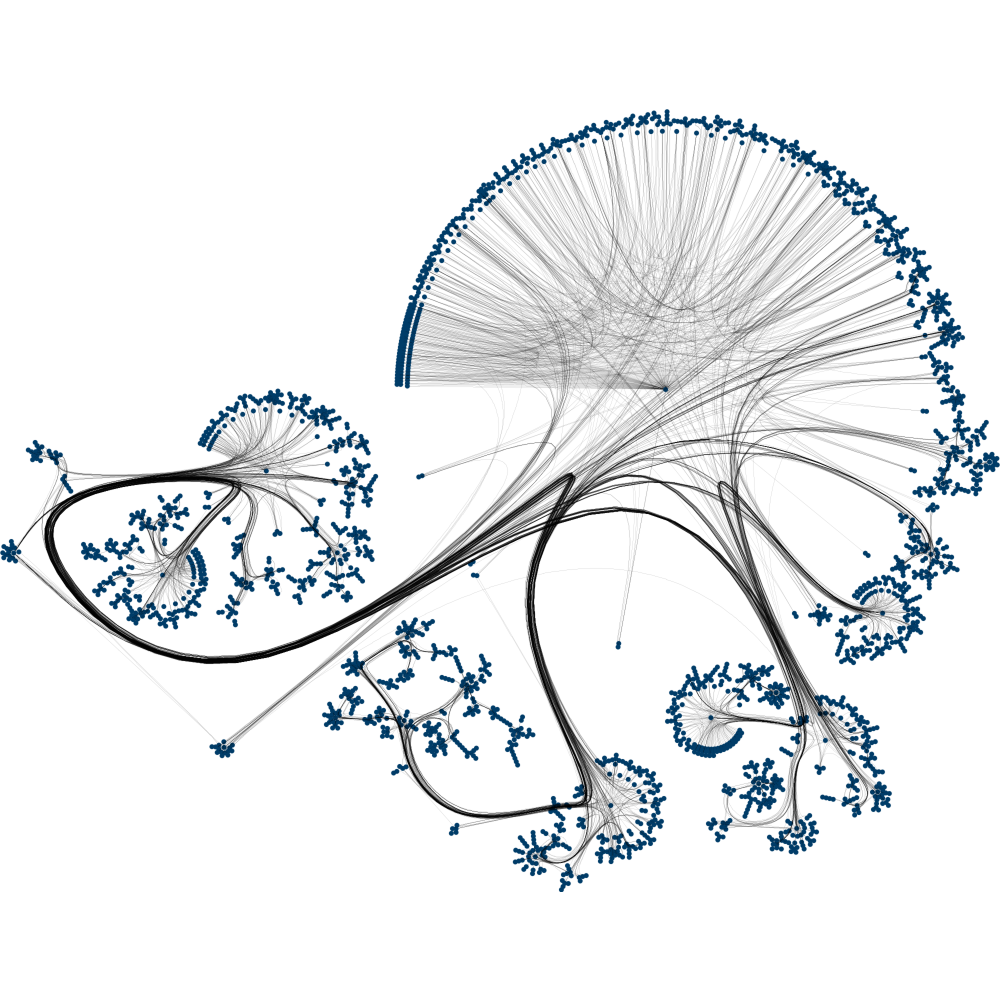# Geodesics

$$k_{\infty, \kappa, \sigma^2}^{(d_g)}(x,x') = \sigma^2\exp\del{-\frac{d_g(x,x')^2}{2\kappa^2}}$$

Theorem. (Feragen et al.) Let $M$ be a complete Riemannian manifold without boundary. If $k_{\infty, \kappa, \sigma^2}^{(d_g)}$ is positive semi-definite for all $\kappa$, then $M$ is isometric to a Euclidean space.

# Stochastic Partial Differential Equations

$$\htmlData{class=fragment,fragment-index=0}{ \underset{\t{Matérn}}{\undergroup{\del{\frac{2\nu}{\kappa^2} - \Delta}^{\frac{\nu}{2}+\frac{d}{4}} f = \c{W}}} }$$ $\Delta$: Laplacian $\c{W}$: Gaussian white noise

# The generalization

$$\htmlData{class=fragment,fragment-index=0}{ \del{\frac{2\nu}{\kappa^2} - \Delta}^{\frac{\nu}{2}+\frac{d}{4}} f = \c{W} }$$

Riemannian Manifolds Undirected Graphs
• $\Delta$ — Laplace–Beltrami, • $\Delta$ — graph Laplacian,
• $\c{W}$ — white noise controlled by the Riemannian volume, • $\c{W}$ — i.i.d. Gaussians,
• $\left( \frac{2 \nu}{\kappa^2} - \Delta \right)^{\frac{\nu}{2} + \frac{d}{4}}$ is defined via functional calculus

# Matérn Kernels on Riemannian Manifolds

Define $\underbrace{H^s(M)}_{\text{Sobolev space}} = \underbrace{(1-\Delta)^{-\frac{s}{2}}}_{\text{Bessel potential}} L^2(M)$

Then the solution of $$\bigg(\frac{2\nu}{\kappa^2} - \Delta\bigg)^{\frac{\nu}{2}+\frac{d}{4}} f = \c{W}$$

At least the solution of $$\bigg(1 - \Delta\bigg)^{\frac{\nu}{2}+\frac{d}{4}} f = \c{W}$$

May be regarded as the isonormal proces on $H^{\nu + d/2}$.
It has the reproducing kernel of $H^{\nu + d/2}$ as its kernel.

# Solution for Compact Riemannian Manifolds

The solution is a Gaussian process with kernel $$\htmlData{class=fragment}{ k_{\nu, \kappa, \sigma^2}(x,x') = \frac{\sigma^2}{C_\nu} \sum_{n=0}^\infty \del{\frac{2\nu}{\kappa^2} - \lambda_n}^{-\nu-\frac{d}{2}} f_n(x) f_n(x') }$$

# Matérn Kernels: Compact Riemannian Manifolds

The solution is a Gaussian process with kernel $$\htmlData{class=fragment}{ k_{\nu, \kappa, \sigma^2}(x,x') = \frac{\sigma^2}{C_\nu} \sum_{n=0}^\infty \del{\frac{2\nu}{\kappa^2} - \lambda_n}^{-\nu-\frac{d}{2}} f_n(x) f_n(x') }$$

## $\lambda_n, f_n$: eigenvalues and eigenfunctions of the Laplace–Beltrami

Proof. 1. Show that the covariance function of the solution is the reproducing kernel of the Sobolev space $H^{\nu + d/2}$.

2. The reproducing kernel may be computed via the Fourier method (known).

# Riemannian Matérn kernels: examples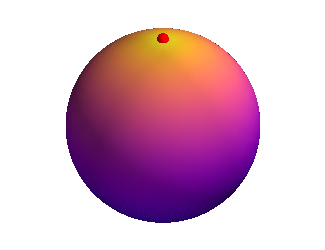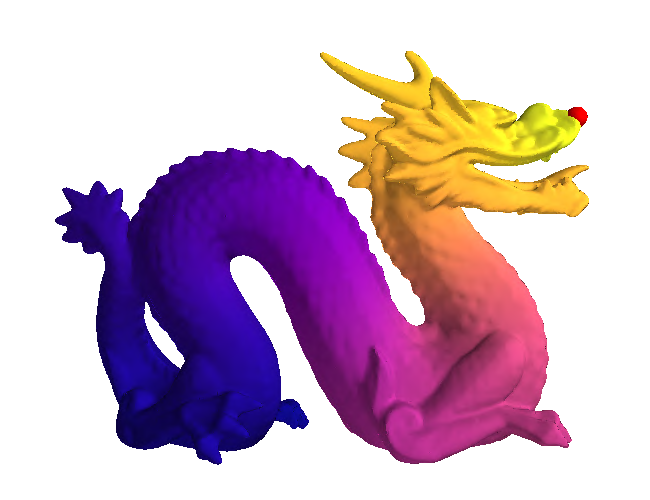# Matérn Kernels: Finite Undirected Graphs

The solution is a Gaussian process with kernel $$\htmlData{class=fragment}{ k_{\nu, \kappa, \sigma^2}(i, j) = \frac{\sigma^2}{C_{\nu}} \sum_{n=0}^{\abs{V}-1} \del{\frac{2\nu}{\kappa^2} + \mathbf{\lambda_n}}^{-\nu} \mathbf{f_n}(i)\mathbf{f_n}(j) }$$

## $\lambda_n, \mathbf{f_n}$: eigenvalues and eigenvectors of the Laplacian matrix# Graph Matérn kernels: examples# Summary

We now can compute kernels on### Manifolds### Graphs

Thus we can use Gaussian process regression on such spaces!

# An Application: Modeling Dynamical Systems with Uncertainty

$$\htmlData{fragment-index=0,class=fragment}{ x_0 } \qquad \htmlData{fragment-index=1,class=fragment}{ x_1 = x_0 + f(x_0)\Delta t } \qquad \htmlData{fragment-index=2,class=fragment}{ x_2 = x_1 + f(x_1)\Delta t } \qquad \htmlData{fragment-index=3,class=fragment}{ .. }$$

# Pendulum Dynamics: GP on a cylinder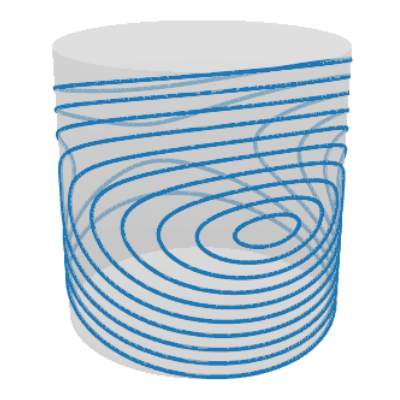### (a) Ground truth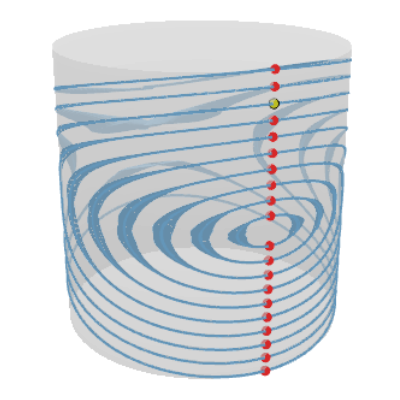# Bayesian Optimization in Robotics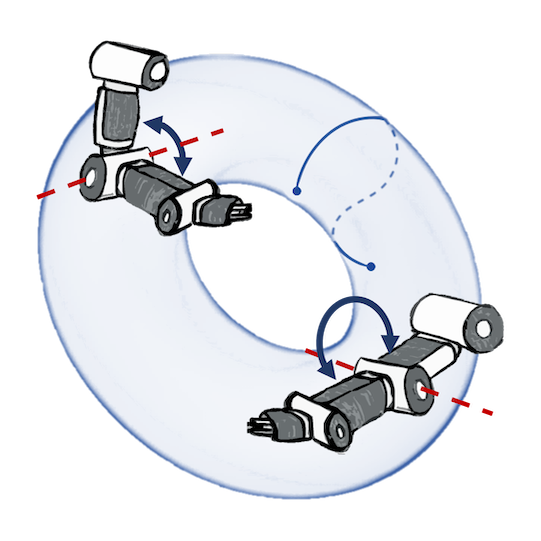### Joint postures: $\mathbb{T}^d$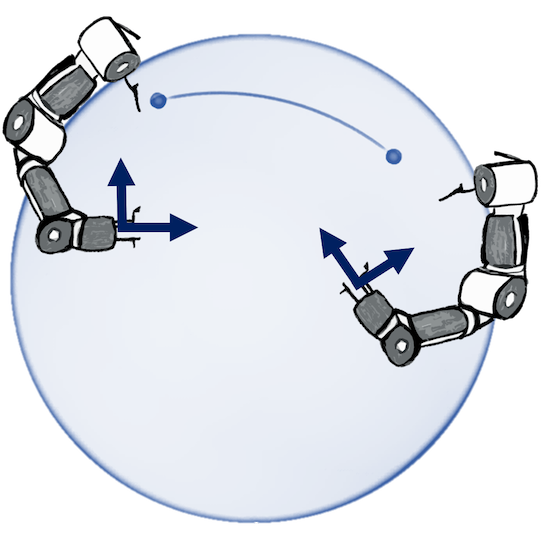### Rotations: $\mathbb{S}^3$, $\operatorname{SO}(3)$# Traffic Speed Extrapolation on a Road Network### (a) Prediction# Wind Speed Modeling: Vector Fields on Manifolds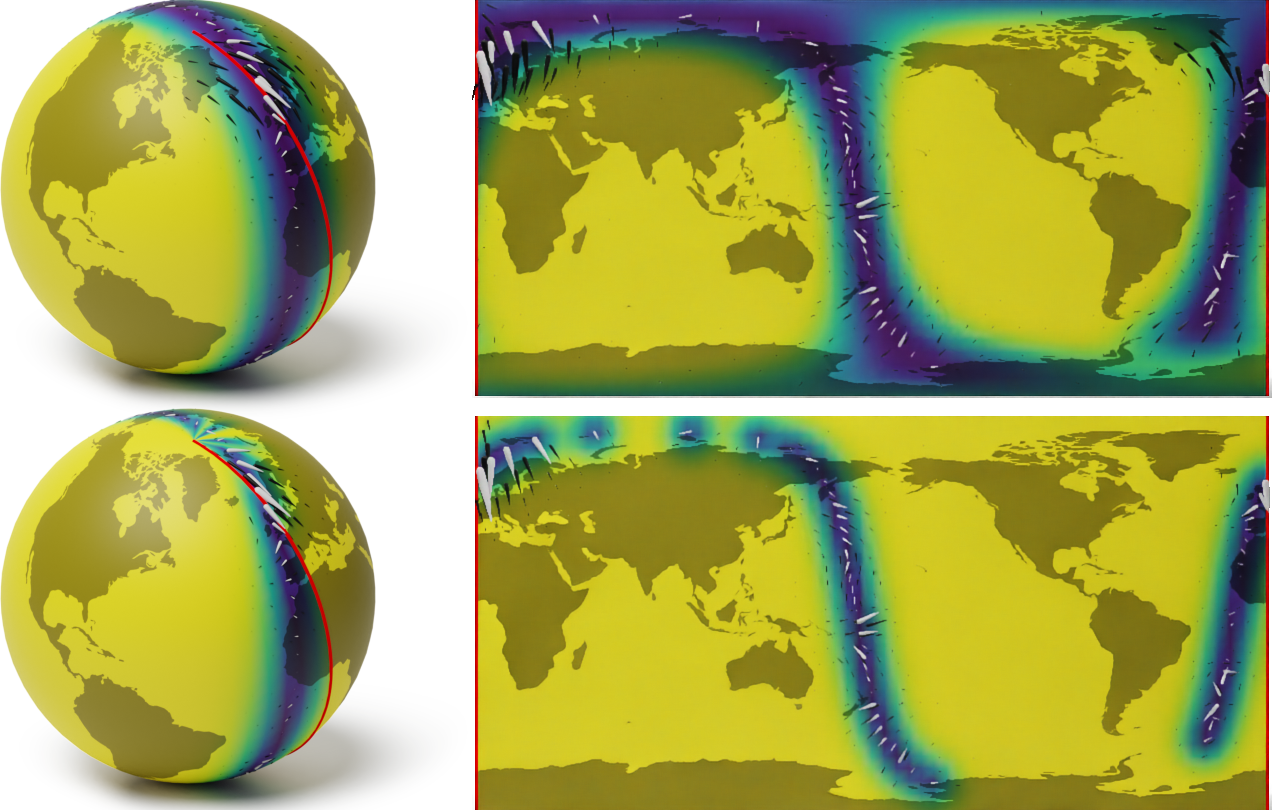# InfoBAX: Shortest Paths for an Unknown Graph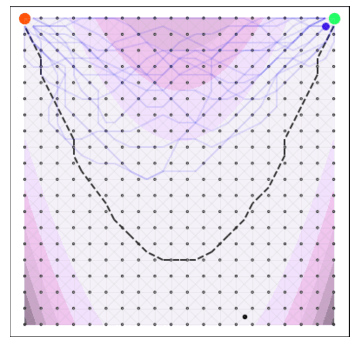# Wind Speed Modeling: Vector Fields on Manifolds

A naive Euclidean model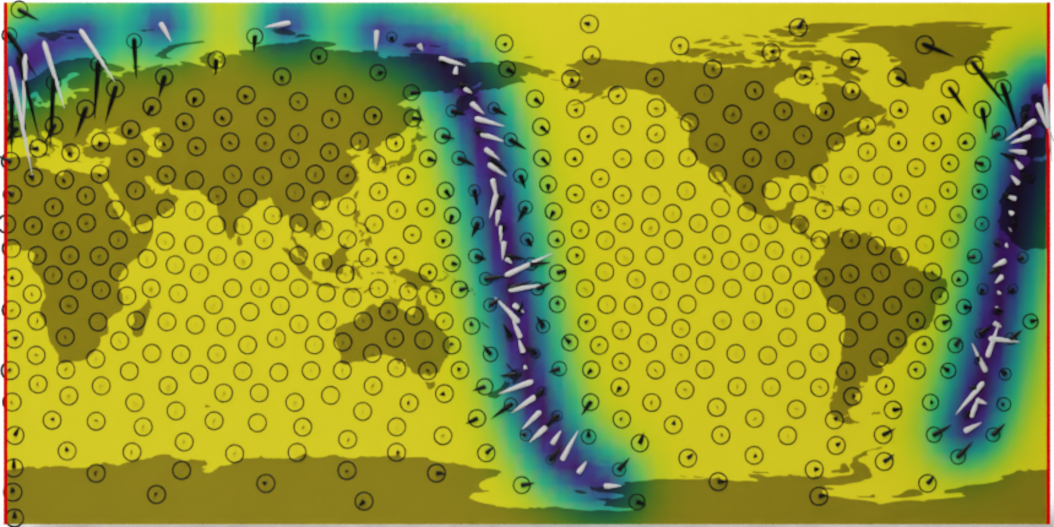Wind speed extrapolation on a map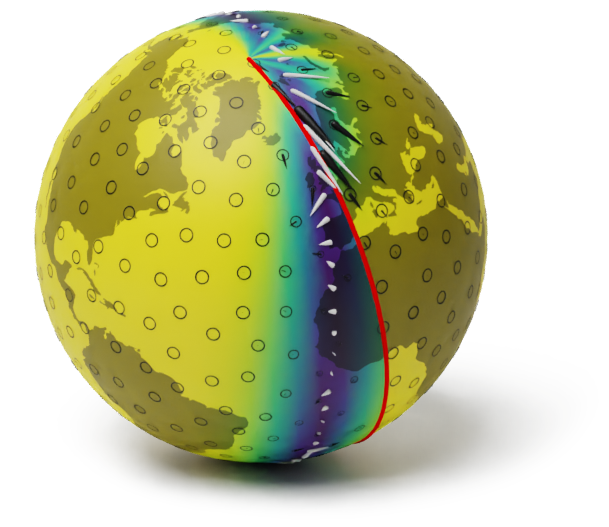The corresponding model on a globe

# Wind Speed Modeling: Vector Fields on Manifolds

A geometry-aware model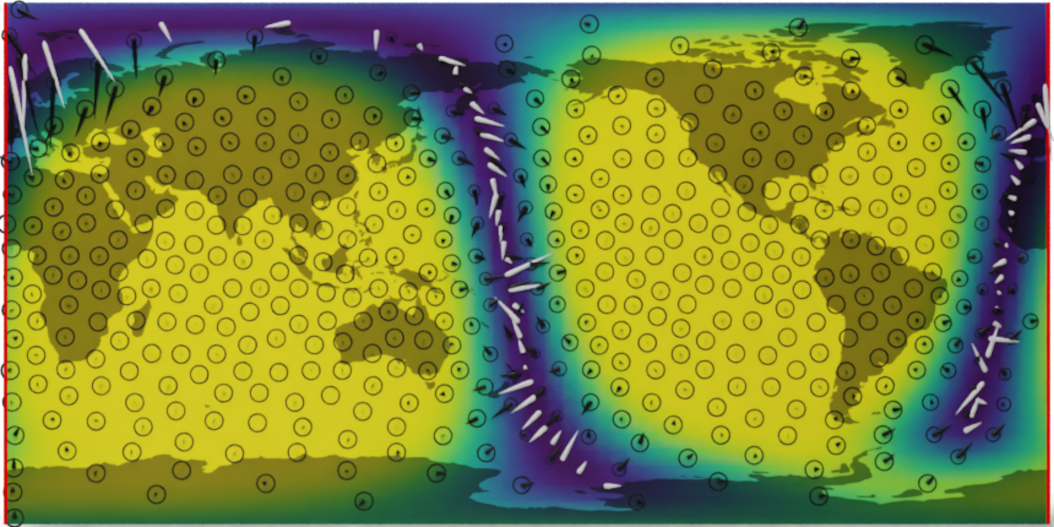Wind speed extrapolation on a map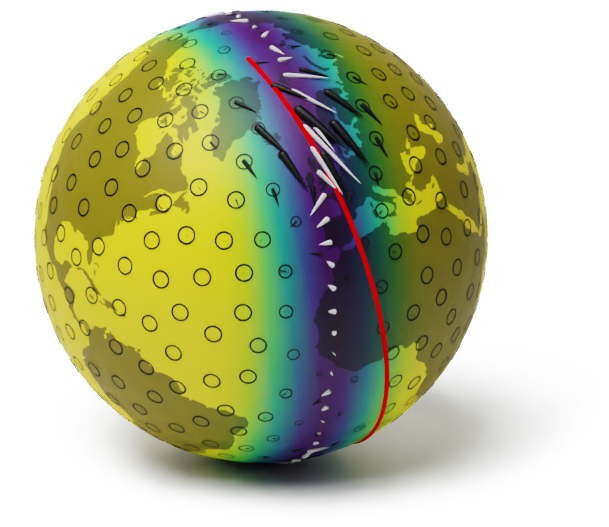The corresponding model on a globe

# Posterior Moments in Terms of the RKHS

Interpolation: $f \given f(\v{x}) = \v{y}$$\hat{m} = \argmin\limits_{f \in \c{H}_k} \norm{f}_{\c{H}_k}$ subject to $f(x_i) = y_i$

$(\hat{m}(x) - f_{\text{true}}(x))^2 \leq \norm{f_{\text{true}}}_{\c{H}_k}^2 \hat{k}(x, x)$

Regression: $f \given f(\v{x}) + \v{\varepsilon} = \v{y}~~$ where $~~\v{\varepsilon} \sim \f{N}(0, \sigma_n^2 I)$$\hat{m} = \argmin\limits_{f \in \c{H}_k} \sum\limits_{i=1}^n (f(x_i) - y_i)^2 + \sigma_n^2 \norm{f}_{\c{H}_k}$

$(\hat{m}(x) - f_{\text{true}}(x))^2 \leq \norm{f_{\text{true}}}_{\c{H}_{k^{\sigma}}}^2 \! \del{\hat{k}(x, x) \!+\! \sigma_n^2}$
Here $\c{H}_{k^{\sigma}} = \c{H}_{k} + \c{H}_{\sigma_n^2 \delta}$ and $x \not= x_i, i = 1, \ldots, n$

# Posterior Moments in Terms of the RKHS: Summary

Moments $\hat{m}(x)$ and $\hat{k}(x, x)$ of the posterior Gaussian process
may be expressed in terms of the RKHS $\c{H}_k$.

# Samples and the RKHS

Consider a covariance $k$ living on a compact space $\c{X}$ with a finite measure $\nu$.

A generalized Driscol’s Zero-One Law. Consider $f \sim \f{GP}(0, k)$ and $r$ such that $\c{H}_k \subseteq \c{H}_r$. Let $I_{k r}: \c{H}_k \to \c{H}_r$ be the natural inclusion operator.

• If $I_{k r}$ is Hilbert-Schmidt, then $f \in \c{H}_r$ with probability $1$.
• Otherwise, $f \in \c{H}_r$ with probability $0$.

Thus $f \in \c{H}_k$ with probability $0$, however...

For any $0 < \theta < 1$ we have with probability $1$: $f \in (\c{H}_k, L^2(\c{X}, \nu))_{\theta, 2}$.

# The reference# Coauthors# PeterMostowsky# IskanderAzangulov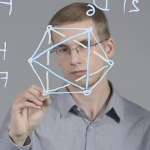# AndreiSmolensky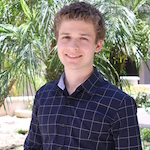# AlexanderTerenin# Marc PeterDeisenroth# SoTakao# Coauthors# NicolasDurrande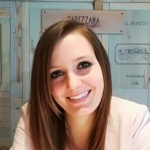# NoémieJaquier# TamimAsfour# LeonelRozo# MichaelHutchinson# Yee WhyTeh# Thank you!

viacheslav.borovitskiy@gmail.com                       https://vab.im# References

Own works:

V. Borovitskiy, A. Terenin, P. Mostowsky, M. P. Deisenroth. Matérn Gaussian Processes on Riemannian Manifolds.
In Neural Information Processing Systems (NeurIPS) 2020.

V. Borovitskiy, I. Azangulov, A. Terenin, P. Mostowsky, M. P. Deisenroth. Matérn Gaussian Processes on Graphs.
In International Conference on Artificial Intelligence and Statistics (AISTATS) 2021.

Other works:

M. Kanagawa, P. Hennig, D. Sejdinovic, B. K. Sriperumbudur. Gaussian Processes and Kernel Methods: A Review on Connections and Equivalences. arXiv preprint arXiv:1807.02582, 2018.

I. Steinwart. Convergence Types and Rates in Generic Karhunen-Loeve Expansions with Applications to Sample Path Properties. Potential Analysis, 2018.

F. Lindgren, H. Rue, J. Lindström. An explicit link between Gaussian fields and Gaussian Markov random fields: the stochastic partial differential equation approach. Journal of the Royal Statistical Society: Series B, 2011.

A. Feragen, F. Lauze, S. Hauberg. Geodesic exponential kernels: When curvature and linearity conflict. Proceedings of the IEEE conference on computer vision and pattern recognition, 2015.

P. Whittle. On Stationary Processes in the Plane. Biometrika, 1954.

viacheslav.borovitskiy@gmail.com                       https://vab.im